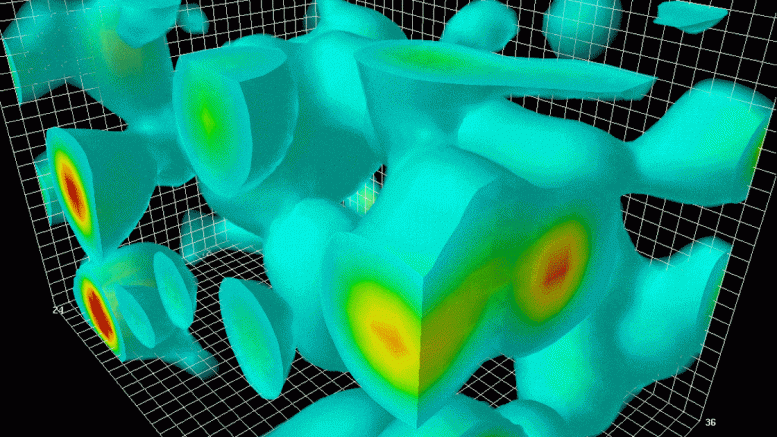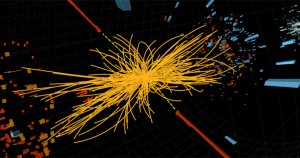# The Formulations of Quantum Field TheoryLattice QCD is a non-perturbative method to approximate the workings of gluon fields around quarks found inside of protons and neutrons | Source: Argonne National Laboratory

Recent developments in modern physics revolve heavily on the notion of a Quantum Universe, with both matter and forces being described as quantized fundamental particles. These particles have set values of experimentally determined mass, electric charge, color charge, spin, and various other characteristics and are organized into the Standard Model of Particle Physics (SM). This modern understanding of the fundamental interactions of the physical world is now categorized into a branch of physics known as Quantum Field Theory (QFT).

The Development of Quantum Mechanics

For the vast majority of scientific history, Classical Mechanics, as described by Newton (and mathematically reformulated various times by Lagrange, Hamilton, and Jacobi), was used to explain the workings of all the questions physics had to offer. In the late 17th century, Maxwell released his papers describing the field of Classical Electrodynamics, tying together both the macroscopic and microscopic physical worlds. Certain inexplicable behaviors in Newtonian Mechanics, such as the reasoning behind Mercury’s orbital variations, were eventually explained by Einstein’s theories of Special and General Relativity (SR, GR). It seemed that although the expanses of physics were slowing down, there were increasingly small numbers of physical occurrences left to explain.Newton’s first law states that an object at rest will stay at rest and that an object in motion will stay in motion unless acted on by a net force. Mathematically this is equivalent to saying that if the net force (depicted with by $$\Sigma \mathbf{F}$$ to show summation) on an object is zero, then the change in velocity $$(\mathbf{v} = \dot{\mathbf{x}})$$ with respect to time is zero $$(\mathbf{a}=\ddot{\mathbf{x}}=0)$$ | Source: Harvard Physics

However, there remained one major flaw in this classical understanding of the Universe: it required matter to be continuously divisible no matter the scale at which these divisions would occur. Gradually, enormous amounts of evidence against a notion of continuous matter were collected, with technological advancements allowing for the earliest understanding of fundamental particles. This idea includes electrons, discovered by Thomson, and the photoelectric effect (photons being quantized discrete chunks or “packets” of light), discovered by Einstein and Planck. This indicated that for a complete theory describing all of the Universe’s fundamental interactions to be formed, the final theory must innately take into account quantum theory.

Unlike Classical physical theories, Quantum Mechanics (QM) no longer dealt with a deterministic view of reality, with the behavior of individual particles being probabilistic. This “wave-particle duality” troubled physicists, as the exact meaning of such a wave-particle relationship was difficult to comprehend. This relationship, along with Heisenberg’s Uncertainty Principle, indicated that the behavior of quanta simply could not be described classically, nor could QM be derived from previous classical equations. QM follows a set of postulates described by the Schrödinger equation, which mathematically represented these various intricacies in the form of its Hermitian operators.The Schrödinger equation is a linear partial differential equation that acts as a postulate for the entirety of Quantum Mechanics. It shows the temporal evolution of a term known as the wave function $$(| \psi (t) \rangle )$$, which describes the position of a particle in a probabilistic wave. Oftentimes, the notation for the position wave function is denoted $$\psi (x)$$, while the time-dependent wave function is denoted $$\Psi (x,t)$$. | Source: Griffiths Introduction to Quantum Mechanics

However, the Time-Dependent Schrödinger Equation and its quantum mechanical operators were not Lorentz invariant. That is to say, the earliest formulations of Quantum Mechanics could not successfully be combined with Einstein’s Special Relativity, as the dependence on time of the quantum operators could not be transferred into other reference frames using the Lorentz Transformation. Eventually, the Klein-Gordon and Dirac equations (relativistic reformulations of  Schrödinger’s equation), as well as incrementally better approximations for the relations between particles at speeds slow relative to the speed of light, allowed physicists to truly pursue QM to its fullest.

Motivations for a Quantum Field Theory

For various phenomena (interactions with small velocities and momenta), it is mathematically legitimate to neglect Einstein’s postulates of Special Relativity, and this led to non-relativistic QM having immense success in predicting certain quantum behaviors. However, it cannot be the final theory in the end, as it does not take into account these relativistic tendencies. Similarly, the Klein-Gordon and Dirac equations, despite being Lorentz invariant, cannot permit a quantum-mechanical description of certain fields.The energy trails of particle physics experiments conducted at the LHC under the collaboration of both ATLAS and CMS. This particular signature included evidence of the existence of the Higgs Boson in 2012 | Source: CERN

The development of particle accelerators allowed for greater support for QM theories. Modern particle physics experiments, such as those being conducted at CERN’s Large Hadron Collider, involve colliding particles together at enormously high energies $$($$$$13\text{ TeV}$$$$)$$, allowing for data to be collected on various characteristics of the signal produced by certain theoretical particles. However, these high energies also mean high velocities (approaching speeds near the speed of light), requiring a Lorentz invariant system of formalization. Also, these high-energy scattering experiments are affected by the various physical fields acting on the particles.

This meant a quantum mechanical theory needed to be formed that could fulfill the postulates of both Special Relativity and experimental evidence that pointed towards fields that were also fundamentally quantum in nature. This theory, or rather the framework of conceptual and mathematical physics, is dubbed Quantum Field Theory. Within this framework exists various Quantum Field Theories, describing various fields and their quantum counterparts, such as Quantum Electrodynamics (QED) and Quantum Chromodynamics (QCD).

Canonical Quantization

One common formulation for procuring a mathematical representation of these quantized fields is by taking the existing classical field theory and then using the Dirac or Klein-Gordon equations to quantize them. The terminology “canonical” refers to the specific classical theory that is used. As in both Hamiltonian classical mechanics, the system’s dynamics are represented using canonical Poisson brackets. However, once the Hamiltonian commutators are quantized, the Poisson brackets are only partially conserved, meaning that this “translation” approach allows for a very close mathematical representation. In a few select instances, the new quantum commutators cannot truly act as an apt translation of all of the fields initial qualities. The canonical quantization method aims to find a suitable description of a quantum field, while still keeping the fundamental nature of the equivalent classical field intact. Otherwise, the QFT would not be able to describe the interactions at both a microscopic and macroscopic scale.To better visualize the interactions of these subatomic particles, Feynman Diagrams are used, simplifying the heavy and often conceptually juxtaposing mathematical concepts, into fairly intuitive diagrams representing certain collisions and scatterings of elementary particles | Source: Forbes

Path Integral Formulation

The path-integral formulation of QFT, as completed by Richard Feynman, revolves around using a Lagrangian rather than a Hamiltonian formulation of Energy relations. This formulation analyzes the various paths a particle could take within a field, and sets them all equal as a weighted sum of these various outcomes, resulting in a final approximation of the path of each particle known as the “probability amplitude.” This can then be set proportional to an exponentiated action given by the Lagrangian. The path-integral method for solving for QFT characteristics seems almost entirely different from the canonical quantization, focusing more on how the particles within the fields move (and therefore, indirectly explaining the behavior of a quantized field). However, despite the vastly contrasting mathematical approaches, the results are equivalent.

Computational Methods

Oftentimes in both Quantum Mechanics and Quantum Field Theories, the mathematical approaches above (generally dubbed “Perturbative Formulations” of QFT) cannot be solved analytically, yielding some deficiencies in how accurate they are theoretically. These shortcomings are appeased with various computational methods, such as Lattice Gauge Theories in Quantum Chromodynamics. These alternative methods are still approximations, but by employing the use of massive supercomputers, the interactions of these particles in a simulated field can be created, allowing for further advancements in physicists’ ability to understand how they behave.

For example, the muon’s magnetic moment g-factor was predicted in 2018 and based on existing Standard Model data, which allowed for experimental differences to be noticed in Fermilab’s most recent run of Muon g-2. Computational methods have been growing in popularity as technological developments in the computing world have progressed, with the quantum computing industry posing the potential for better representing such a massive scale of interactions in a brief amount of time.